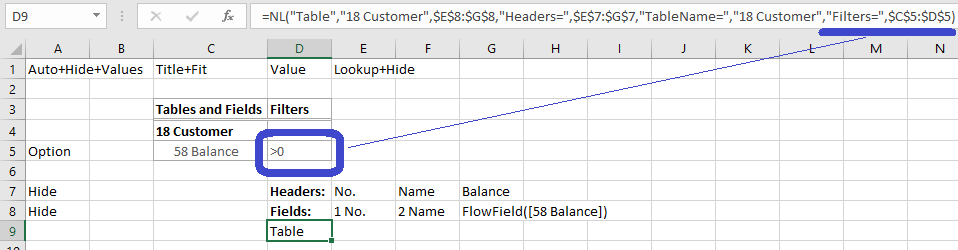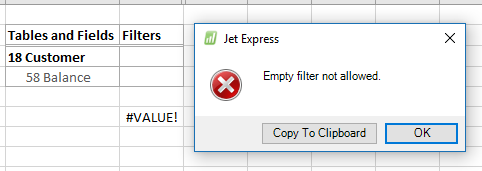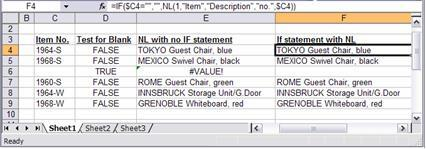Community
Training
Submit a Request
Become a Jet Insider
Give Feedback

# Blank Filters - using @@ and other techniques

Help Topics...

### Overview

Sometimes you want to filter an NL function based on the contents of another cell or the output of another NL function, where either of which could be a blank value.

The NL function does not allow you to use blank filters, and you will get a #VALUE error message if you fill in a FilterField parameter and leave the corresponding filter blank.

One example of this is an NL("Table") function which includes user-defined report option:If the user were to leave the Balance filter blank, an error would result:### Examples

1. An example of this would be retrieving an Item Description based on the output of an NL function which that lists Item numbers from a Sales Invoices table.

You could have Sales Invoices which do not have item numbers, so the NL fucntion would return a blank value.  This is where you need to use Excel's IF function.

Assume that an NL function is listing item numbers in cell C4.  If cell C4 is blank, you want cell D4 to be blank, too.  Otherwise, you want to filter an NL function in D4 based on the contents of C4.

The first step is to test cell C4 to see if it is blank. Excel will do that for you using the following formula.

C4=""

The formula above uses two double quote characters to represent a blank cell and gives you a True/False response.

NL ("First","Item","Description","No.",C4)

#### Combining the two functions above into an IF function gives the following result.

`=IF(C4="","",NL("First","Item","Description","No.",C4))`

The spreadsheet below has the results of the formulas described above.2. The example above assumes that blank values are not something you want to find.

Sometimes you actually do want to find all records that have a blank value.  In this case, you can use two single quotes ('').

In the above example, if C4 could be blank and you wanted to use it as a filter anyway, you could use the following formula.

=NL("First","Item","Description","No.","'"&C4&"'")

Although this formula is a little hard to read, it is putting a single quote character (') on either side of the C4 reference.  The & function is an Excel function that combines two strings of text into one, so the result of this formula will be the value in cell C4 with a single quote character on either side of it.  If C4 contains the Item No. of Shampoo1, the resulting filter will be Shampoo1.  If C4 is blank, the result is (two single quotes), which is a valid NAV filter for blank fields.

#### An easier to read variation on the filter for blanks is "@@" in front of the value in C4.  The function becomes the following.

`=NL("First","Item","Description","No.","@@"&C4)`